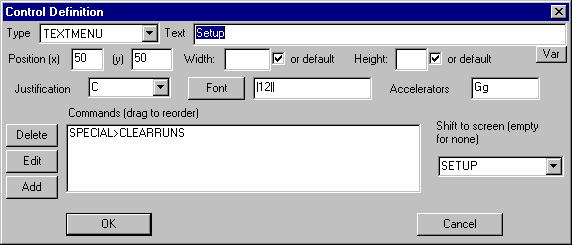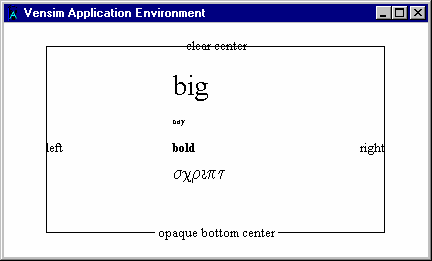# Vensim Help

You can make an active link to data contained in a spreadsheet using the GET 123 DATA, GET DIRECT DATA or GET XLS DATA function.  For example suppose you have a spreadsheet file:and want to use the data in row 2.  Then you can write an equation

sales data := GET XLS DATA('test6.xls','sheet1','1','A2') ~~|

After simulating the model setting Print Every on the table tool to 10 we would get:The sales data  variable could then be used anywhere in the model to drive behavior.  For subscripted models you can define a vector of data series in this manner using a single equation.